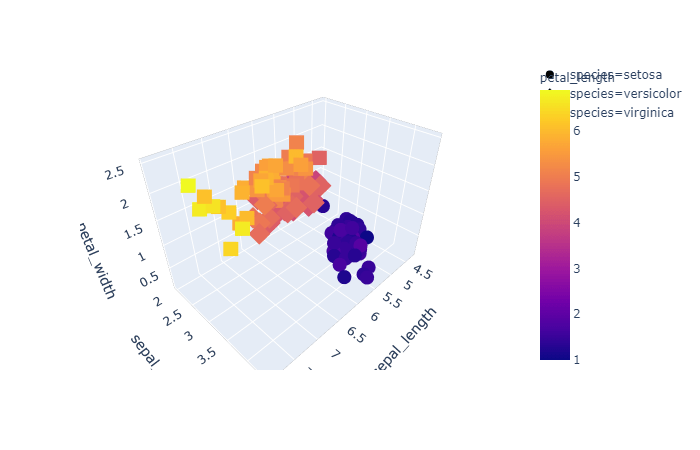# Handling missing data in pandas data frame python

In this post we are going to discuss how to handle missing data from a pandas data frame. Find total number of missing data in the data frame missing_total = df.isnull().sum().sum() Find number of missing data in each column in a data frame missing_per_column = df.isnull().sum() Investigate patterns in the amount of missing data in … Continue reading Handling missing data in pandas data frame python# Print ROC AUC Receiver Operating Characteristic Area Under Curve

The receiver operating characteristic area under curve is a way to measure the performance of a classification model, may be created using algorithms like Logistic Regression. ROC-AUC is basically a graph where we plot true positive rate on y-axis and false positive rate on x-axis. If a model is good the AUC will be close to 1. Area … Continue reading Print ROC AUC Receiver Operating Characteristic Area Under Curve# Visualize and Print Confusion Matrix

In many cases you would like to print the confusion matrix in a better format and look and feel than what is provided by scikit learn by default. The default look when printing confusion matrix using scikit learn scikit-learn default confusion matrix print However in many cases you may like to print the confusion matrix … Continue reading Visualize and Print Confusion Matrix# What is Confusion Matrix in Machine Learning

Not only human beings but also the machine learning models may get confused !! After all Artificial Intelligence mimics a human brain, isn’t it?(pun intended). Imagine yourself as a machine learning engineer and suppose you trained a machine learning classification model successfully today. After the model is trained you checked the accuracy which is 93.0%. Wow … Continue reading What is Confusion Matrix in Machine Learning# Scatter Plot using Matplotlib in Python

A scatter plot is a type of plot or graph that uses two coordinates axis (say x and y) to display values for two variables for a set of data. We can use matplotlib library of python to create scatter plot in Jupyter notebook. Let us import the required libraries first: #Import the libraries import … Continue reading Scatter Plot using Matplotlib in Python# How to Create 3d Interactive Graph using Plotly

Like matplotlib and seaborn we have plotly, that is a data visualization library used for creating graphical, 3d and interactive graph. You can install plotly using below command pip install plotly Then you can import it in Jupyter notebook as below. import plotly.express as pex Let us use the famous iris dataset to create the … Continue reading How to Create 3d Interactive Graph using Plotly

# Scatter Plot using Lmplot Function of Seaborn

Apart from the methods scatterplot and regplot, seaborn also provides lmplot as another function to draw a scatterplot. However when we create scatter plots using seaborn’s lmplot, it will introduce a regression line in the plot. Let us first import libraries and load the data required to create the plot. import numpy as np import … Continue reading Scatter Plot using Lmplot Function of Seaborn

# Scatter Plot using Regplot Function of Seaborn

Though we have an obvious method named, scatterplot, provided by seaborn to draw a scatterplot, seaborn provides other methods as well to draw scatter plot. One of the other method is regplot. However when we create scatter plots using seaborn's regplot method, it will introduce a regression line in the plot as regplot is based … Continue reading Scatter Plot using Regplot Function of Seaborn